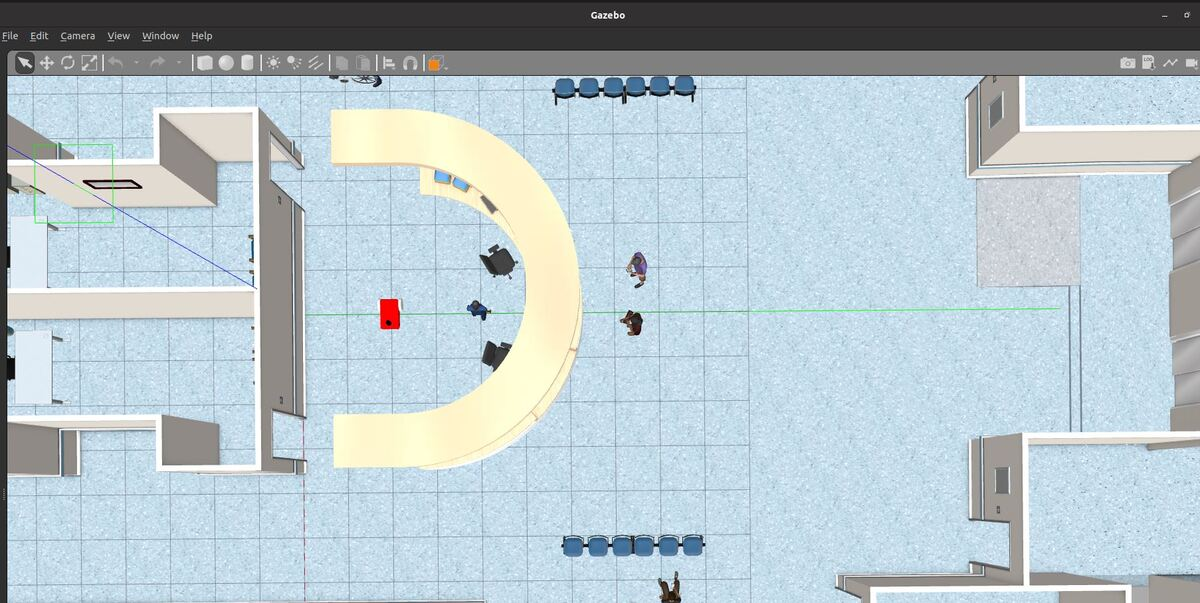# How to Create a tf Listener Using ROS 2 and PythonLet’s write a node that will display the coordinate transformation between the map frame and the base_link frame. We will listen to the transform, convert the transform to 2D format (x, y, yaw angle), and then publish the 2D format to a topic.

# Prerequisites

You can find the files for this post here on my Google Drive.

# Directions

The way to do to a coordinate transformation manually is to run this command:

`ros2 run tf2_ros tf2_echo map base_link`

The syntax is:

`ros2 run tf2_ros tf2_echo [parent_frame] [child_frame]`

The command above gives you the pose of the child frame inside the parent frame.

You will often hear the parent_frame called the reference_frame. The child_frame is often called the target_frame.

Open a terminal window, and type the following command.

`cd ~/dev_ws/`

Install the relevant packages (I’m assuming you have ROS 2 Galactic. If you have another ROS 2 distribution, replace ‘galactic’ with the name of that distribution).

`sudo apt-get install ros-galactic-turtle-tf2-py ros-galactic-tf2-tools ros-galactic-tf-transformations`
`pip3 install transforms3d`
`pip3 install numpy`
`cd ~/dev_ws/src/two_wheeled_robot/scripts`

Open a new Python script.

`mkdir transforms`
`cd transforms`
`gedit map_to_base_link_transform.py`
```#!/usr/bin/env python3

"""
Description:
Publish the coordinate transformation between the map frame
The output is [x,y,yaw]. yaw is -pi to pi
-------
Subscription Topics:
/tf - geometry_msgs/TransformStamped[]
-------
Publishing Topics:
-------
Date: November 25, 2021
"""

# Import the ROS client library for Python
import rclpy

# Enables the use of rclpy's Node class
from rclpy.node import Node

# Base class to handle exceptions
from tf2_ros import TransformException

# Stores known frames and offers frame graph requests
from tf2_ros.buffer import Buffer

# Easy way to request and receive coordinate frame transform information
from tf2_ros.transform_listener import TransformListener

# Handle float64 arrays
from std_msgs.msg import Float64MultiArray

# Math library
import math

class FrameListener(Node):
"""
Subclass of the Node class.
The class listens to coordinate transformations and
publishes the 2D pose at a specific time interval.
"""
def __init__(self):
"""
Class constructor to set up the node
"""

# Initiate the Node class's constructor and give it a name

# Declare and acquire `target_frame` parameter
self.target_frame = self.get_parameter(
'target_frame').get_parameter_value().string_value

self.tf_buffer = Buffer()
self.tf_listener = TransformListener(self.tf_buffer, self)

# Create publisher(s)

# This node publishes the 2d pose.
# Maximum queue size of 1.
self.publisher_2d_pose = self.create_publisher(
Float64MultiArray,
1)

# Call on_timer function on a set interval
timer_period = 0.1
self.timer = self.create_timer(timer_period, self.on_timer)

# Current position and orientation of the target frame with respect to the
# reference frame. x and y are in meters, and yaw is in radians.
self.current_x = 0.0
self.current_y = 0.0
self.current_yaw = 0.0

def on_timer(self):
"""
Callback function.
This function gets called at the specific time interval.
"""
# Store frame names in variables that will be used to
# compute transformations
from_frame_rel = self.target_frame
to_frame_rel = 'map'

trans = None

try:
now = rclpy.time.Time()
trans = self.tf_buffer.lookup_transform(
to_frame_rel,
from_frame_rel,
now)
except TransformException as ex:
self.get_logger().info(
f'Could not transform {to_frame_rel} to {from_frame_rel}: {ex}')
return

# Publish the 2D pose
self.current_x = trans.transform.translation.x
self.current_y = trans.transform.translation.y
roll, pitch, yaw = self.euler_from_quaternion(
trans.transform.rotation.x,
trans.transform.rotation.y,
trans.transform.rotation.z,
trans.transform.rotation.w)
self.current_yaw = yaw
msg = Float64MultiArray()
msg.data = [self.current_x, self.current_y, self.current_yaw]
self.publisher_2d_pose.publish(msg)

def euler_from_quaternion(self, x, y, z, w):
"""
Convert a quaternion into euler angles (roll, pitch, yaw)
roll is rotation around x in radians (counterclockwise)
pitch is rotation around y in radians (counterclockwise)
yaw is rotation around z in radians (counterclockwise)
"""
t0 = +2.0 * (w * x + y * z)
t1 = +1.0 - 2.0 * (x * x + y * y)
roll_x = math.atan2(t0, t1)

t2 = +2.0 * (w * y - z * x)
t2 = +1.0 if t2 > +1.0 else t2
t2 = -1.0 if t2 < -1.0 else t2
pitch_y = math.asin(t2)

t3 = +2.0 * (w * z + x * y)
t4 = +1.0 - 2.0 * (y * y + z * z)
yaw_z = math.atan2(t3, t4)

return roll_x, pitch_y, yaw_z # in radians

def main(args=None):

# Initialize the rclpy library
rclpy.init(args=args)

# Create the node
frame_listener_node = FrameListener()

# Spin the node so the callback function is called.
# Publish any pending messages to the topics.
try:
rclpy.spin(frame_listener_node)
except KeyboardInterrupt:
pass

# Shutdown the ROS client library for Python
rclpy.shutdown()

if __name__ == '__main__':
main()
```

Save the file, and close it.

Now let’s update our CMakeLists.txt file.

`cd ~/dev_ws/src/two_wheeled_robot`
`gedit CMakeLists.txt`

Build the file.

`cd ~/dev_ws/`
`colcon build`

Launch a robot. I will use this command from this tutorial. Everything below is a single command.

`ros2 launch two_wheeled_robot hospital_world_connect_to_charging_dock.launch.py`

Open another terminal and run the transform listener.

`ros2 run two_wheeled_robot map_to_base_link_transform.py`

Ignore the messages that are printed to the terminal (e.g. Could not transform … the earliest data is at time …).

The command above does the base_link -> map transform. If you want to see another transform (e.g. lidar_link -> map), you can type the following command:

`ros2 run two_wheeled_robot map_to_base_link_transform.py --ros-args -p target_frame:='lidar_link'`

Open a new terminal window, and observe the data.

`ros2 topic echo /map_to_base_link_pose2d`

Here we can see the pose of the robot (i.e. base_link coordinate frame) with respect to the map frame in x, y, yaw (i.e. Euler angle) format…where x and y are in meters, and yaw is in radians.

In a real-world scenario, if you have an object that is 2 meters in front of the robot, for example, its pose with respect to the base_link would be (x=2.0, y=0.0, yaw=0.0). And then to get the pose of the object with respect to the map frame, we would add those coordinates to the output of the base_link to map transform above.